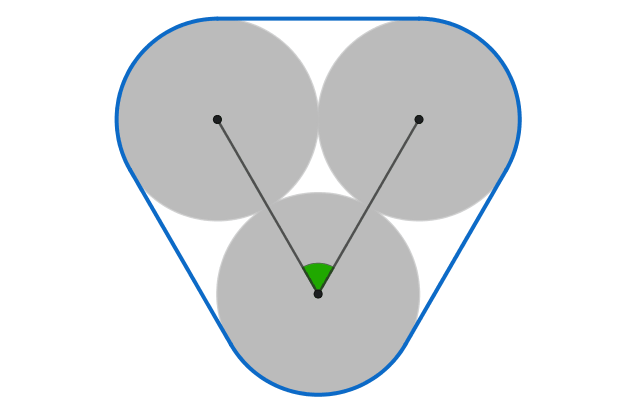# 3 coins

Calculus Level 3The centers of three identical coins form the angle that's colored green above.

What angle maximizes the area of the blue convex hull?

Note: You can imagine the perimeter of the convex hull as a rubber band stretched around the three coins.

×